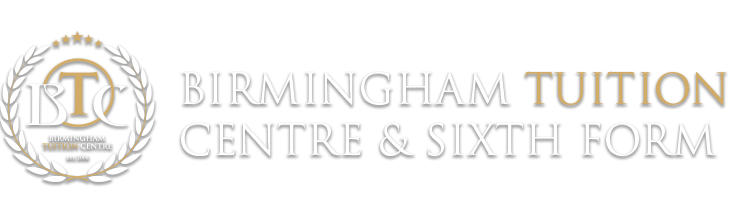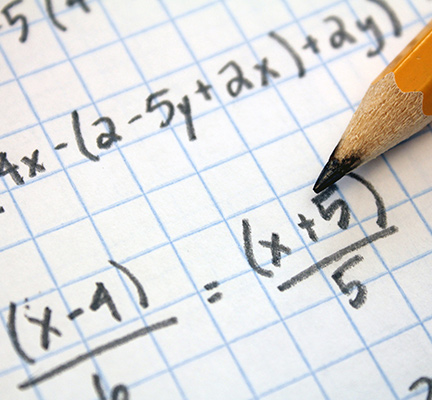## GCSE Mathematics“Mathematics is the queen of science, and arithmetic the queen of mathematics.”Carl Friedrich Gauss

GCSE Mathematics is a challenging subject which enables students to think logically and apply a wide range of analytical techniques to real life problems. It is a prerequisite for all Universities and a compulsory qualification for the majority of employers.

Approximately 60% of employers state that they encounter difficulty in finding and recruiting people with adequate mathematical skills. In view of this, BTC deems it essential that students continually develop their mathematical skills and attain the highest possible grade in their Mathematics GCSE.

This enhances their future job prospects and also allows students to establish themselves as keen Mathematicians.

Our GCSE Mathematics course comprises the following modules:

Topic Exemplar Question Students Are Expected To
Number Work out: 2/5 × 7/8

· Perform calculations with integers

· Understand whole number theory

· Combine arithmetic operations

·Perform inverse operations

·Perform calculations with fractions and percentages

·Solve simple problems related to direct and inverse proportion

Algebra Simplify the following expression:
7x2 + 7x + 3 – 4x + x2

· Use Algebra to construct proofs and arguments

· Simplify algebraic products and quotients using the laws of indices

· Solve simultaneous equations

· Interpret the language of functions

Geometry State the formula used to calculate the area of triangle.

· Familiarise themselves with geometrical skills, conventions, notation and terms

· Solve plane geometry problems using X and Y coordinates

·Recall and use properties of angles

Measures What formula is used to calculate the surface area of sphere?

· Convert measurements from one unit to another

· Use and interpret maps and scale drawings

· Understand and use bearings

· Perform area, volume and surface area calculations

Statistics Take a look at the table below showing data about the number of people in cars.

Number of people in a car

1

2

3

4

5

Frequency

15

6

3

1

0

What’s the mean number of people found in a car?

· Understand and use statistical problem solving process/handling data cycle

· Calculate estimates of mean, median, mode, range of grouped data

· Interpret and construct diagrams for grouped data.

· Analyse data to find patterns and exceptions

Probability Fiza has 10 coins in a bag. There are three £1 coins and seven 50 pence coins. Fiza takes at random, 3 coins from the bag. Work out the probability that she takes exactly £2.50

· Understand and use the vocabulary of probability and probability scale

·Compare experimental data and theoretical probabilities

·Understand and use estimates or measures of probability from theoretical models (including equally likely outcomes), or from relative frequency